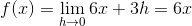﻿ Calculus - YoosFuhl.com

# Calculus

### 1. Rates of change

rate of change of function f on x = a is: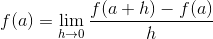### 2. Derivative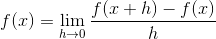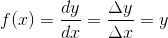Example:
Derivative of f(x) = 3x² + 1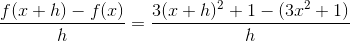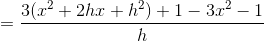= 3 (2x + h)

= 6x + 3h